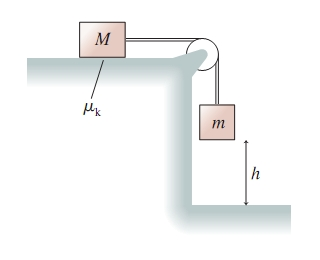# Problem: Use work and energy to find an expression for the speed of the block in the following figure just before it hits the floor.(a) Find an expression for the speed of the block if the coefficient of kinetic friction for the block on the table is μk.(b) Find an expression for the speed of the block if the table is frictionless.

###### FREE Expert Solution

In this problem, we are asked to use work-energy relationship.

$\overline{){\mathbf{W}}{\mathbf{=}}{\mathbf{∆}}{\mathbf{K}}{\mathbf{E}}}$

ΔK = Kf - Ki

W = -ΔU + Wnc where Wnc is the work done by non-conservative forces in the path.

85% (463 ratings)###### Problem Details

Use work and energy to find an expression for the speed of the block in the following figure just before it hits the floor.(a) Find an expression for the speed of the block if the coefficient of kinetic friction for the block on the table is μk.

(b) Find an expression for the speed of the block if the table is frictionless.

Frequently Asked Questions

What scientific concept do you need to know in order to solve this problem?

Our tutors have indicated that to solve this problem you will need to apply the Energy in Connected Objects (Systems) concept. You can view video lessons to learn Energy in Connected Objects (Systems). Or if you need more Energy in Connected Objects (Systems) practice, you can also practice Energy in Connected Objects (Systems) practice problems.

What professor is this problem relevant for?

Based on our data, we think this problem is relevant for Professor Pantziris' class at UTAH.